Tamil Nadu Board of Secondary EducationSSLC (English Medium) Class 10th
Advertisement Remove all ads

# Samacheer Kalvi solutions for Mathematics Class 10 SSLC Tamil Nadu State Board chapter 4 - Geometry [Latest edition]

Advertisement Remove all ads

#### Chapters## Chapter 4: Geometry

Exercise 4.1Exercise 4.2Exercise 4.3Exercise 4.4Exercise 4.5Unit Exercise – 4
Exercise 4.1 [Pages 170 - 171]

### Samacheer Kalvi solutions for Mathematics Class 10 SSLC Tamil Nadu State Board Chapter 4 Geometry Exercise 4.1 [Pages 170 - 171]

Exercise 4.1 | Q 1. (i) | Page 170

Check whether the triangles are similar and find the value of x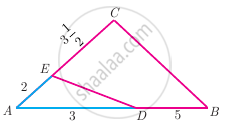Exercise 4.1 | Q 1. (ii) | Page 170

Check whether the triangles are similar and find the value of x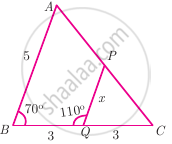Exercise 4.1 | Q 2 | Page 170

A girl looks the reflection of the top of the lamp post on the mirror which is 6.6 m away from the foot of the lamppost. The girl whose height is 1.25 m is standing 2.5 m away from the mirror. Assuming the mirror is placed on the ground facing the sky and the girl, mirror and the lamppost are in the same line, find the height of the lamp post.

Exercise 4.1 | Q 3 | Page 170

A vertical stick of length 6 m casts a shadow 400 cm long on the ground and at the same time a tower casts a shadow 28 m long. Using similarity, find the height of the tower

Exercise 4.1 | Q 4 | Page 170

Two triangles QPR and QSR, right angled at P and S respectively are drawn on the same base QR and on the same side of QR. If PR and SQ intersect at T, prove that PT × TR = ST × TQ

Exercise 4.1 | Q 5 | Page 170

In the adjacent figure, ∆ABC is right angled at C and DE ⊥ AB. Prove that ∆ABC ~ ∆ADE and hence find the lengths of AE and DE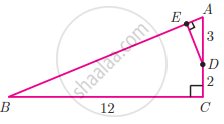Exercise 4.1 | Q 6 | Page 170

In the adjacent figure, ∆ACB ~ ∆APQ. If BC = 8 cm, PQ = 4 cm, BA = 6.5 cm and AP = 2.8 cm, find CA and AQ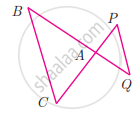Exercise 4.1 | Q 7. (i) | Page 171

If figure OPRQ is a square and ∠MLN = 90°. Prove that ∆LOP ~ ∆QMO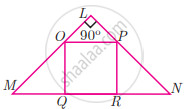Exercise 4.1 | Q 7. (ii) | Page 171

If figure OPRQ is a square and ∠MLN = 90°. Prove that ∆LOP ~ ∆RPNExercise 4.1 | Q 7. (iii) | Page 171

If figure OPRQ is a square and ∠MLN = 90°. Prove that ∆QMO ~ ∆RPNExercise 4.1 | Q 7. (iv) | Page 171

If figure OPRQ is a square and ∠MLN = 90°. Prove that QR2 = MQ × RNExercise 4.1 | Q 8 | Page 171

If ∆ABC ~ ∆DEF such that area of ∆ABC is 9 cm2 and the area of ∆DEF is 16 cm2 and BC = 2.1 cm. Find the length of EF.

Exercise 4.1 | Q 9 | Page 171

Two vertical poles of heights 6 m and 3 m are erected above a horizontal ground AC. Find the value of y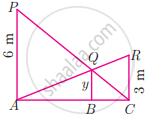Exercise 4.1 | Q 10 | Page 171

Construct a triangle similar to a given triangle PQR with its sides equal to 2/3 of the corresponding sides of the triangle PQR (scale factor 2/3 < 1)

Exercise 4.1 | Q 11 | Page 171

Construct a triangle similar to a given triangle LMN with its sides equal to 4/5 of the corresponding sides of the triangle LMN (scale factor 4/5 < 1)

Exercise 4.1 | Q 12 | Page 171

Construct a triangle similar to a given triangle ABC with its sides equal to 6/5 of the corresponding sides of the triangle ABC (scale factor 6/5 > 1)

Exercise 4.1 | Q 13 | Page 171

Construct a triangle similar to a given triangle PQR with its sides equal to 7/3 of the corresponding sides of the triangle PQR (scale factor 7/3 > 1)

Advertisement Remove all ads
Exercise 4.2 [Pages 181 - 183]

### Samacheer Kalvi solutions for Mathematics Class 10 SSLC Tamil Nadu State Board Chapter 4 Geometry Exercise 4.2 [Pages 181 - 183]

Exercise 4.2 | Q 1. (i) | Page 181

In ∆ABC, D and E are points on the sides AB and AC respectively such that DE || BC

If "AD"/"DB" = 3/4 and AC = 15 cm find AE

Exercise 4.2 | Q 1. (ii) | Page 181

In ∆ABC, D and E are points on the sides AB and AC respectively such that DE || BC

If AD = 8x – 7, DB = 5x – 3, AE = 4x – 3 and EC = 3x – 1, find the value of x

Exercise 4.2 | Q 2 | Page 182

ABCD is a trapezium in which AB || DC and P, Q are points on AD and BC respectively, such that PQ || DC if PD = 18 cm, BQ = 35 cm and QC = 15 cm, find AD

Exercise 4.2 | Q 3. (i) | Page 182

In ΔABC, D and E are points on the sides AB and AC respectively. For the following case show that DE || BC

AB = 12 cm, AD = 8 cm, AE = 12 cm and AC = 18 cm

Exercise 4.2 | Q 3. (ii) | Page 182

In ΔABC, D and E are points on the sides AB and AC respectively. For the following case show that DE || BC

AB = 5.6 cm, AD = 1.4 cm, AC = 7.2 cm and AE = 1.8 cm.

Exercise 4.2 | Q 4. (i) | Page 182

If PQ || BC and PR || CD prove that "AR"/"AD" = "AQ"/"AB"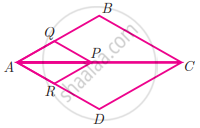Exercise 4.2 | Q 4. (ii) | Page 182

If PQ || BC and PR || CD prove that "QB"/"AQ" = "DR"/"AR"Exercise 4.2 | Q 5 | Page 182

Rhombus PQRB is inscribed in ΔABC such that ∠B is one of its angle. P, Q and R lie on AB, AC and BC respectively. If AB = 12 cm and BC = 6 cm, find the sides PQ, RB of the rhombus.

Exercise 4.2 | Q 6 | Page 182

In trapezium ABCD, AB || DC, E and F are points on non-parallel sides AD and BC respectively, such that EF || AB. Show that = "AE"/"ED" = "BF"/"FC"

Exercise 4.2 | Q 7 | Page 182

DE || BC and CD || EE Prove that AD2 = AB × AF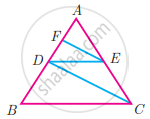Exercise 4.2 | Q 8. (i) | Page 182

Check whether AD is bisector of ∠A of ∆ABC of the following
AB = 5 cm, AC = 10 cm, BD = 1.5 cm and CD = 3.5 cm

Exercise 4.2 | Q 8. (ii) | Page 182

Check whether AD is bisector of ∠A of ∆ABC of the following

AB = 4 cm, AC = 6 cm, BD = 1.6 cm and CD = 2.4 cm.

Exercise 4.2 | Q 9 | Page 182

∠QPR = 90°, PS is its bisector. If ST ⊥ PR, prove that ST × (PQ + PR) = PQ × PR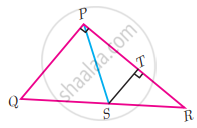Exercise 4.2 | Q 10 | Page 182

ABCD is a quadrilateral in which AB = AD, the bisector of ∠BAC and ∠CAD intersect the sides BC and CD at the points E and F respectively. Prove that EF || BD.

Exercise 4.2 | Q 11 | Page 182

Construct a ∆PQR in which the base PQ = 4.5 cm, ∠R = 35° and the median from R to RG is 6 cm.

Exercise 4.2 | Q 12 | Page 182

Construct a ∆PQR in which QR = 5 cm, ∠P = 40° and the median PG from P to QR is 4.4 cm. Find the length of the altitude from P to QR.

Exercise 4.2 | Q 13 | Page 182

Construct a ∆PQR such that QR = 6.5 cm, ∠P = 60° and the altitude from P to QR is of length 4.5 cm

Exercise 4.2 | Q 14 | Page 182

Construct a ∆ABC such that AB = 5.5 cm, ∠C = 25° and the altitude from C to AB is 4 cm

Exercise 4.2 | Q 15 | Page 183

Draw a triangle ABC of base BC = 5.6 cm, ∠A = 40° and the bisector of ∠A meets BC at D such that CD = 4 cm

Exercise 4.2 | Q 16 | Page 183

Draw ∆PQR such that PQ = 6.8 cm, vertical angle is 50° and the bisector of the vertical angle meets the base at D where PD = 5.2 cm

Advertisement Remove all ads
Exercise 4.3 [Pages 187 - 188]

### Samacheer Kalvi solutions for Mathematics Class 10 SSLC Tamil Nadu State Board Chapter 4 Geometry Exercise 4.3 [Pages 187 - 188]

Exercise 4.3 | Q 1 | Page 187

A man goes 18 m due east and then 24 m due north. Find the distance of his current position from the starting point?

Exercise 4.3 | Q 2 | Page 187

There are two paths that one can choose to go from Sarah’s house to James's house. One way is to take C street, and the other way requires to take B street and then A street. How much shorter is the direct path along C street?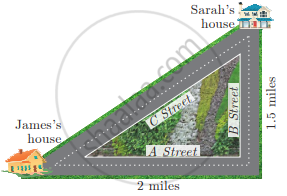Exercise 4.3 | Q 3 | Page 187

To get from point A to point B you must avoid walking through a pond. You must walk 34 m south and 41 m east. To the nearest meter, how many meters would be saved if it were possible to make a way through the pond?

Exercise 4.3 | Q 4 | Page 187

In the rectangle WXYZ, XY + YZ = 17 cm, and XZ + YW = 26 cm. Calculate the length and breadth of the rectangle?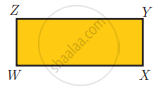Exercise 4.3 | Q 5 | Page 187

The hypotenuse of a right triangle is 6 m more than twice of the shortest side. If the third side is 2 m less than the hypotenuse, find the sides of the triangle

Exercise 4.3 | Q 6 | Page 187

5 m long ladder is placed leaning towards a vertical wall such that it reaches the wall at a point 4 m high. If the foot of the ladder is moved 1.6 m towards the wall, then find the distance by which the top of the ladder would slide upwards on the wall.

Exercise 4.3 | Q 7 | Page 188

The perpendicular PS on the base QR of a ∆PQR intersects QR at S, such that QS = 3 SR. Prove that 2PQ2 = 2PR2 + QR2

Exercise 4.3 | Q 8 | Page 188

In the adjacent figure, ABC is a right angled triangle with right angle at B and points D, E trisect BC. Prove that 8AE2 = 3AC2 + 5AD2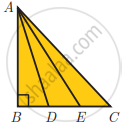Advertisement Remove all ads
Exercise 4.4 [Pages 198 - 199]

### Samacheer Kalvi solutions for Mathematics Class 10 SSLC Tamil Nadu State Board Chapter 4 Geometry Exercise 4.4 [Pages 198 - 199]

Exercise 4.4 | Q 1 | Page 198

The length of the tangent to a circle from a point P, which is 25 cm away from the centre is 24 cm. What is the radius of the circle?

Exercise 4.4 | Q 2 | Page 198

∆LMN is a right angled triangle with ∠L = 90°. A circle is inscribed in it. The lengths of the sides containing the right angle are 6 cm and 8 cm. Find the radius of the circle.

Exercise 4.4 | Q 3 | Page 198

A circle is inscribed in ∆ABC having sides 8 cm, 10 cm and 12 cm as shown in the figure, Find AD, BE and CF.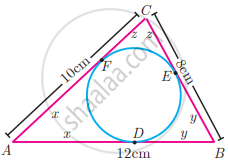Exercise 4.4 | Q 4 | Page 198

PQ is a tangent drawn from a point P to a circle with centre O and QOR is a diameter of the circle such that ∠POR = 120°. Find ∠OPQ.

Exercise 4.4 | Q 5 | Page 198

A tangent ST to a circle touches it at B. AB is a chord such that ∠ABT = 65°. Find ∠AOB, where “O” is the centre of the circle.

Exercise 4.4 | Q 6 | Page 198

O is the centre of the circle with radius 5 cm. T is a point such that OT = 13 cm and OT intersects the circle E, if AB is the tangent to the circle at E, find the length of AB.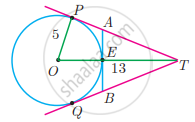Exercise 4.4 | Q 7 | Page 198

In two concentric circles, a chord of length 16 cm of larger circle becomes a tangent to the smaller circle whose radius is 6 cm. Find the radius of the larger circle

Exercise 4.4 | Q 8 | Page 198

Two circles with centres O and O’ of radii 3 cm and 4 cm, respectively intersect at two points P and Q, such that OP and O’P are tangents to the two circles. Find the length of the common chord PQ.

Exercise 4.4 | Q 9 | Page 198

Show that the angle bisectors of a triangle are concurrent

Exercise 4.4 | Q 10 | Page 198

An artist has created a triangular stained glass window and has one strip of small length left before completing the window. She needs to figure out the length of left out portion based on the lengths of the other sides as shown in the figure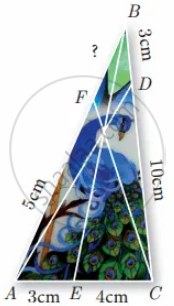Exercise 4.4 | Q 11 | Page 198

Draw a tangent at any point R on the circle of radius 3.4 cm and centre at P?

Exercise 4.4 | Q 12 | Page 198

Draw a circle of radius 4.5 cm. Take a point on the circle. Draw the tangent at that point using the alternate segment theorem

Exercise 4.4 | Q 13 | Page 198

Draw the two tangents from a point which is 10 cm away from the centre of a circle of radius 5 cm. Also, measure the lengths of the tangents.

Exercise 4.4 | Q 14 | Page 198

Take a point which is 11 cm away from the centre of a circle of radius 4 cm and draw the two tangents to the circle from that point.

Exercise 4.4 | Q 15 | Page 198

Draw the two tangents from a point which is 5 cm away from the centre of a circle of diameter 6 cm. Also, measure the lengths of the tangents.

Exercise 4.4 | Q 16 | Page 199

Draw a tangent to the circle from the point P having radius 3.6 cm, and centre at O. Point P is at a distance 7.2 cm from the centre.

Advertisement Remove all ads
Exercise 4.5 [Pages 199 - 200]

### Samacheer Kalvi solutions for Mathematics Class 10 SSLC Tamil Nadu State Board Chapter 4 Geometry Exercise 4.5 [Pages 199 - 200]

#### Multiple choice questions

Exercise 4.5 | Q 1 | Page 199

If in triangles ABC and EDF, "AB"/"DE" = "BC"/"FD" then they will be similar, when

• ∠B = ∠E

• ∠A = ∠D

• ∠B = ∠D

• ∠A = ∠F

Exercise 4.5 | Q 2 | Page 199

In ∆LMN, ∠L = 60°, ∠M = 50°. If ∆LMN ~ ∆PQR then the value of ∠R is

• 40°

• 70°

• 30°

• 110°

Exercise 4.5 | Q 3 | Page 199

If ∆ABC is an isosceles triangle with ∠C = 90° and AC = 5 cm, then AB is

• 2.5 cm

• 5 cm

• 10 cm

• 5sqrt(2)  "cm"

Exercise 4.5 | Q 4 | Page 199

ST || QR, PS = 2 cm and SQ = 3 cm. Then the ratio of the area of ∆PQR to the area of ∆PST is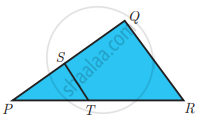• 25 : 4

• 25 : 7

• 25 : 11

• 25 : 13

Exercise 4.5 | Q 5 | Page 199

The perimeters of two similar triangles ∆ABC and ∆PQR are 36 cm and 24 cm respectively. If PQ = 10 cm, then the length of AB is

• 6 2/3 cm

• (10sqrt(6))/3 cm

• 66 2/3 cm

• 15 cm

Exercise 4.5 | Q 6 | Page 199

If in ∆ABC, DE || BC. AB = 3.6 cm, AC = 2.4 cm and AD = 2.1 cm then the length of AE is

• 1.4 cm

• 1.8 cm

• 1.2 cm

• 1.05 cm

Exercise 4.5 | Q 7 | Page 199

In a ∆ABC, AD is the bisector of ∠BAC. If AB = 8 cm, BD = 6 cm and DC = 3 cm. The length of the side AC is

• 6 cm

• 4 cm

• 3 cm

• 8 cm

Exercise 4.5 | Q 8 | Page 199

In the adjacent figure ∠BAC = 90° and AD ⊥ BC then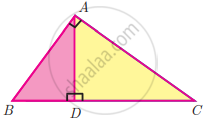• BD.CD = BC

• AB.AC = BC

• BD.CD = AD

• AB.AC = AD

Exercise 4.5 | Q 9 | Page 199

Two poles of heights 6 m and 11 m stand vertically on a plane ground. If the distance between their feet is 12 m, what is the distance between their tops?

• 13 m

• 14 m

• 15 m

• 12.8 m

Exercise 4.5 | Q 10 | Page 199

PR = 26 cm, QR = 24 cm, ∠PAQ = 90°, PA = 6 cm and QA = 8 cm. Find ∠PQR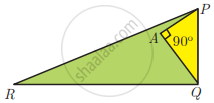• 80°

• 85°

• 75°

• 90°

Exercise 4.5 | Q 11 | Page 200

A tangent is perpendicular to the radius at the

• centre

• point of contact

• infinity

• chord

Exercise 4.5 | Q 12 | Page 200

How many tangents can be drawn to the circle from an exterior point?

• one

• two

• infinite

• zero

Exercise 4.5 | Q 13 | Page 200

The two tangents from an external points P to a circle with centre at O are PA and PB. If ∠APB = 70° then the value of ∠AOB is

• 100°

• 110°

• 120°

• 130°

Exercise 4.5 | Q 14 | Page 200

CP and CQ are tangents to a circle with centre at 0. ARB is another tangent touching the circle at R. If CP = 11 cm and BC = 7 cm, then the length of BR is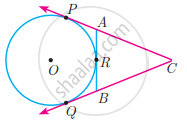• 6 cm

• 5 cm

• 8 cm

• 4 cm

Exercise 4.5 | Q 15 | Page 200

If PR is tangent to the circle at P and O is the centre of the circle, then ∠POQ is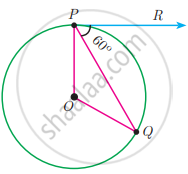• 120°

• 100°

• 110°

• 90°

Advertisement Remove all ads
Unit Exercise – 4 [Pages 200 - 201]

### Samacheer Kalvi solutions for Mathematics Class 10 SSLC Tamil Nadu State Board Chapter 4 Geometry Unit Exercise – 4 [Pages 200 - 201]

Unit Exercise – 4 | Q 1. (i) | Page 200

If BD ⊥ AC and CE ⊥ AB, prove that ∆AEC ~ ∆ADB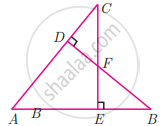Unit Exercise – 4 | Q 1. (ii) | Page 200

If BD ⊥ AC and CE ⊥ AB, prove that "CA"/"AB" = "CE"/"DB"Unit Exercise – 4 | Q 2 | Page 200

In the given figure AB || CD || EF. If AB = 6 cm, CD = x cm, EF = 4 cm, BD = 5 cm and DE = y cm. Find x and y.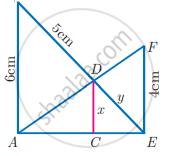Unit Exercise – 4 | Q 3 | Page 200

O is any point inside a triangle ABC. The bisector of ∠AOB, ∠BOC and ∠COA meet the sides AB, BC and CA in point D, E and F respectively. Show that AD × BE × CF = DB × EC × FA

Unit Exercise – 4 | Q 4 | Page 200

ABC is a triangle in which AB = AC. Points D and E are points on the side AB and AC respectively such that AD = AE. Show that the points B, C, E and D lie on a same circle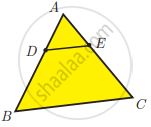Unit Exercise – 4 | Q 5 | Page 200

Two trains leave a railway station at the same time. The first train travels due west and the second train due north. The first train travels at a speed of (20 "km")/"hr" and the second train travels at (30 "km")/"hr". After 2 hours, what is the distance between them?

Unit Exercise – 4 | Q 6. (i) | Page 201

D is the mid point of side BC and AE ⊥ BC. If BC = a, AC = b, AB = c, ED = x, AD = p and AE = h, prove that b2 = "p"^2 + "a"x + "a"^2/4

Unit Exercise – 4 | Q 6. (ii) | Page 201

D is the mid point of side BC and AE ⊥ BC. If BC = a, AC = b, AB = c, ED = x, AD = p and AE = h, prove that c2 = "p"^2 - "a"x + "a"^2/4

Unit Exercise – 4 | Q 6. (iii) | Page 201

D is the mid point of side BC and AE ⊥ BC. If BC = a, AC = b, AB = c, ED = x, AD = p and AE = h, prove that b2 + c2 = 2"p"^2 + "a"^2/2

Unit Exercise – 4 | Q 7 | Page 201

A man whose eye-level is 2 m above the ground wishes to find the height of a tree. He places a mirror horizontally on the ground 20 m from the tree and finds that if he stands at a point C which is 4 m from the mirror B, he can see the reflection of the top of the tree. How height is the tree?

Unit Exercise – 4 | Q 8 | Page 201

An Emu which is 8 feet tall is standing at the foot of a pillar which is 30 feet high. It walks away from the pillar. The shadow of the Emu falls beyond Emu. What is the relation between the length of the shadow and the distance from the Emu to the pillar?

Unit Exercise – 4 | Q 9 | Page 201

Two circles intersect at A and B. From a point, P on one of the circles lines PAC and PBD are drawn intersecting the second circle at C and D. Prove that CD is parallel to the tangent at P.

Unit Exercise – 4 | Q 10 | Page 201

Let ABC be a triangle and D, E, F are points on the respective sides AB, BC, AC or their extensions. Let AD : DB = 5 : 3, BE : EC = 3 : 2 and AC = 21 . Find the length of the line segment CF

Advertisement Remove all ads

## Chapter 4: Geometry

Exercise 4.1Exercise 4.2Exercise 4.3Exercise 4.4Exercise 4.5Unit Exercise – 4## Samacheer Kalvi solutions for Mathematics Class 10 SSLC Tamil Nadu State Board chapter 4 - Geometry

Samacheer Kalvi solutions for Mathematics Class 10 SSLC Tamil Nadu State Board chapter 4 (Geometry) include all questions with solution and detail explanation. This will clear students doubts about any question and improve application skills while preparing for board exams. The detailed, step-by-step solutions will help you understand the concepts better and clear your confusions, if any. Shaalaa.com has the Tamil Nadu Board of Secondary Education Mathematics Class 10 SSLC Tamil Nadu State Board solutions in a manner that help students grasp basic concepts better and faster.

Further, we at Shaalaa.com provide such solutions so that students can prepare for written exams. Samacheer Kalvi textbook solutions can be a core help for self-study and acts as a perfect self-help guidance for students.

Concepts covered in Mathematics Class 10 SSLC Tamil Nadu State Board chapter 4 Geometry are Introduction of Geometry, Similarity, Thales Theorem and Angle Bisector Theorem, Converse of Pythagoras Theorem, Circles and Tangents, Concurrency Theorems, Right-angled Triangles and Pythagoras Property.

Using Samacheer Kalvi Class 10th solutions Geometry exercise by students are an easy way to prepare for the exams, as they involve solutions arranged chapter-wise also page wise. The questions involved in Samacheer Kalvi Solutions are important questions that can be asked in the final exam. Maximum students of Tamil Nadu Board of Secondary Education Class 10th prefer Samacheer Kalvi Textbook Solutions to score more in exam.

Get the free view of chapter 4 Geometry Class 10th extra questions for Mathematics Class 10 SSLC Tamil Nadu State Board and can use Shaalaa.com to keep it handy for your exam preparation

Advertisement Remove all ads
Share
Notifications

View all notifications

Forgot password?